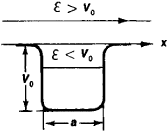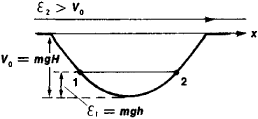# Potential Well

(redirected from Quantum confinement effect)
Also found in: Dictionary, Acronyms.

## potential well

[pə′ten·chəl ¦wel]
(physics)
For an object in a conservative field of force, a region in which the object has a lower potential energy than in all the surrounding regions.

## Potential Well

in physics, a bounded region of space in which the potential energy of a particle is less than that outside the region. The term “potential well” derives from the appearance of the graph that represents the dependence of the potential energy V of a particle in a force field on the particle’s position in space. (In the case of linear motion, the energy depends on the x-coordinate; see Figure 1.) This form of the function V(x) arises in a field of attractive forces. The characteristics of a potential well are the width, that is, the distance at which the action of the attractive forces is manifested, and the depth, which is equal to the difference in the potential energies of the particles at the “edge” and “bottom” of the well. The bottom corresponds to the minimum potential energy. The main property of a potential well is its ability to confine a particle whose total energy ℰ is less than the depth of the well V0; such a particle within a potential well will be in a bound state.Figure 1. Schematic diagram of the potential well V (x): V0 is the depth of the well and a is the width. The total energy £ of a particle is conserved and therefore is represented on the graph by a horizontal line.

In classical mechanics, a particle with energy ℰ < Vo will be unable to escape from the potential well and will always move in the bounded region of the well. The particle’s position at the bottom of the well corresponds to a stable equilibrium and is reached when the particle’s kinetic energy ℰ kin = &— V = 0. If ℰ > Vo, then the particle will overcome the effect of the attractive forces and escape from the well. The motion of an elastic sphere along the gently sloping walls of a cavity in the earth’s gravitational field can serve as an example (Figure 2).Figure 2. A sphere of mass m with energy ℇ1 < V0 cannot escape from the cavity. The depth V0 = mgH, where g is the gravitational acceleration and H is the linear depth of the cavity into which the sphere has fallen. If friction is disregarded, the sphere will oscillate between points 1 and 2, rising only to the height h =1/mg. If the energy of the sphere is ℇ1 > V0, it will escape from the cavity and move toward infinity with a constant velocity ν determined by the relation mv2/2 =2 V0.

In quantum mechanics, in contrast to classical mechanics, the energy of a particle in a bound state in a potential well cai. assume only certain discrete values; that is, there exist discrete energy levels. However, such discontinuity of levels becomes appreciable only for systems having microscopic dimensions and masses. The interval Δ ℰ between energy levels for a particle of mass m in a “deep” well of width a is of the order of the magnitude Δ ℰ ≃ ℏ2/ma2, where ℏ is Planck’s constant. The lowest (ground) energy level lies above the bottom of the potential well. In a well of small depth, that is, V0 ≤ ℏ, a bound state may be absent altogether. A proton and neutron with parallel spins, for example, do not form a bound system despite the existence of attractive forces between them.

Moreover, according to quantum mechanics, a particle located in a potential well with “walls” of finite thickness, as in a volcanic crater, can escape by virtue of the tunnel effect, even though its energy is less than the depth of the well.

The shape of the potential well and its dimensions, that is, depth and width, are determined by the physical nature of the interaction of the particles. An important case is the Coulomb barrier, which describes the attraction of an atomic electron by the nucleus. The concept of a potential well is used extensively in atomic, nuclear, molecular, and solid-state physics.

References in periodicals archive ?
Downscaling the device dimensions has stimulated the extensive efforts to further reduce the gate oxide thickness where a strong quantum confinement effect is expected.
This fact corresponds to an increase of Sinc diameter according to the quantum confinement effect. The crystalline nature of the Sinc was verified by Raman shift measurements as indicated in Figure 4.
On the other hand, the quantum confinement effect is not always observed in practice for Sinc/Si[O.sub.2]-based materials.
Ghadiry, "Quantum confinement effect on trilayer graphene nanoribbon carrier concentration," Journal of Experimental Nanoscience, 2013.
Zelaya-Angel, "Meyer-Neldel-like manifestation of the quantum confinement effect in solid ensembles of semiconductor quantum dots," Physical Review B, vol.
If ZnSe NCs are formed, the emission wavelengths will be less than 443 nm due to the quantum confinement effect. However, we cannot observe the emission peak around or less than 443 nm.
The bandgap of these alloys can also be tuned from near-infrared (IR) to ultraviolet (UV) regimes, and in addition these alloys can be fabricated to further increase their bandgap energy by quantum confinement effects .
In particular, semiconductor nanostructures have received a great deal of attention due to their size-tunable optical properties, quantum confinement effects, and applicability in a wide range of technologies (Henglein, 1989; Steigerwald and Brus, 1990; Alivasatos, 1996).
The quantum confinement effects of these low dimensional materials can be used to achieve high thermoelectric figure of merit by controlling the energy distribution of electrons and phonons independently [1-6].
Nanoscience, the manipulation of matter at the atomic and molecular level, has produced nanomaterials so small their behavior and characteristics deviate from those of macroscopic specimens and maybe predicted by scaling laws or by quantum confinement effects. Writing for postgraduate students of chemistry and materials science and researchers new to nanoscience and nanotechnology, Roduner (physical chemistry, U.
Some researchers attribute the glow to quantum confinement effects in microscopic silicon "wires" left behind after etching: Electrons confined by these wires recombine with positive charges to produce light.

Site: Follow: Share:
Open / Close